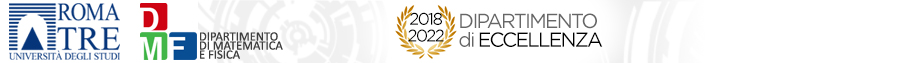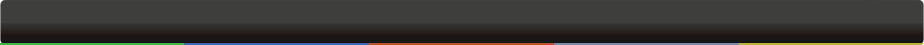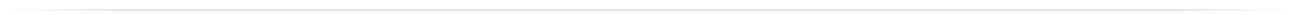# Seminari del Dipartimento

 GeometriaEffective Adjunction TheoryMarco Andreatta 17-10-2019 - 15:45Largo San Leonardo Murialdo,1 - Pal.C - Aula 211 Let X be a projective variety, with canonical divisor K, and H a Cartier divisor on X. The effectivity, or non effectivity, of some adjoint divisors aK + bH, for suitable a,b, determines the geometry of X. I will first give a proof of the following version of the Termination of Adjunction: X with at most canonical singularities is uniruled if and only if for each very ample Cartier divisor H on X we have that mK+H is not effective for some m=m(H)>0. Then I will discuss the following Conjecture: Assume that X has terminal singularities, H is nef and big and s >0. K+tH is not effective for every integer t with 1 ≤ t ≤ s if and only if K+sH is not pseudo-effective; this is true if and only if the pair (X,L) is birational to a precise list of (uniruled) models. The Conjecture is true for s ≥ (dimX-1); this can be proved via the Theory of the Reductions, started by Fujita and Sommese, which nowadays can be interpreted as a Minimal Model Program with Scaling.org: VIVIANI Filippo